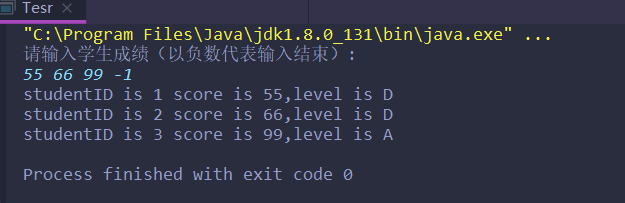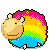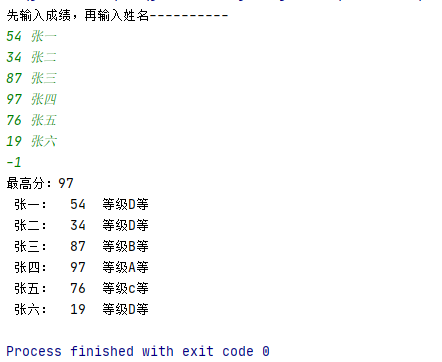• 利用Vector代替数组处理：从键盘读入学生成绩（以负数代表输入结束），找出最高分，并输出学生成绩等级。 提示：数组一旦创建，长度就固定不变，所以在创建数组前就需要知道它的长度。而向量类java.util.Vector可以...
利用Vector代替数组处理：从键盘读入学生成绩（以负数代表输入结束），找出最高分，并输出学生成绩等级。
提示：数组一旦创建，长度就固定不变，所以在创建数组前就需要知道它的长度。而向量类java.util.Vector可以根据需要动态伸缩。
1创建Vector对象：Vector v=new Vector();
2给向量添加元素：v.addElement(obj);   //obj必须是对象
3取出向量中的元素：Object  obj=v.elementAt(0);
4注意第一个元素的下标是0，返回值是Object类型的。
5计算向量的长度：v.size();
若与最高分相差10分内：A等；20分内：B等；30分内：C等；其它：D等

public class WorkVector {
public static void main(String[] args) {
Vector c=new Vector();
int n=0;
int max=0;
System.out.println("请输入学生成绩：");
System.out.println("提示：若输出为负数则录入结束");
while(n>=0){
Scanner input=new Scanner(System.in);
n=input.nextInt();
if(n>=0&&n<=100) {
c.addElement(n);
}
}
System.out.println(c.size());
for(int i=0;i<c.size();i++) {
if(((int)c.elementAt(i))>max) {
max=(int)c.elementAt(i);
}
System.out.println(c.elementAt(i));
}
System.out.println("最高分为："+max);
for(int i=0;i<c.size();i++) {
if(max-((int)c.elementAt(i))<=10) {
System.out.println(c.elementAt(i)+"\tA等");
}else if(max-((int)c.elementAt(i))<=20) {
System.out.println(c.elementAt(i)+"\tB等");
}else if(max-((int)c.elementAt(i))<=30) {
System.out.println(c.elementAt(i)+"\tC等");
}else {
System.out.println(c.elementAt(i)+"\tD等");
}
}
}
}



展开全文• 方法1 :数组方法,不推荐练习用 ...利用Vector代替数组处理(数组处理见下方注释)：从键盘读入学生成绩（以负数代表输入结束），找出 最高分，并输出学生成绩等级。 提示：数组一旦创建，长度就固定不变
方法1 :数组方法,不推荐, 练习数组可用
2. 从键盘读入学生成绩，找出最高分，并输出学生成绩等级。
成绩>=最高分-10 等级为’A’
成绩>=最高分-20 等级为’B’
成绩>=最高分-30 等级为’C’
其余 等级为’D’
提示：先读入学生人数，根据人数创建int数组，
存放学生成绩。
方法2:利用Vector代替数组
利用Vector代替数组处理(数组处理见下方注释)：从键盘读入学生成绩（以负数代表输入结束），找出
最高分，并输出学生成绩等级。
提示：数组一旦创建，长度就固定不变，所以在创建数组前就需要知道它的
长度。而向量类java.util.Vector可以根据需要动态伸缩。
创建Vector对象：Vector v=new Vector();
给向量添加元素：v.addElement(Object obj); //obj必须是对象
取出向量中的元素：Object obj=v.elementAt(0);
注意第一个元素的下标是0，返回值是Object类型的。
计算向量的长度：v.size();
若与最高分相差10分内：A等；20分内：B等；
30分内：C等；其它：D等
方法1,2 代码:
package ScoreGrade;

import sun.reflect.generics.scope.Scope;

import javax.swing.text.StyledEditorKit;
import java.util.Scanner;
import java.util.Vector;

/**
* @version v-1.8.0_131.
* @auther Jack hou  Email:256595662@qq.com
* @data 2021/2/4/11:11
* @Description:
**/
/*方法2:推荐
利用Vector代替数组处理(数组处理见下方注释)：从键盘读入学生成绩（以负数代表输入结束），找出
最高分，并输出学生成绩等级。
提示：数组一旦创建，长度就固定不变，所以在创建数组前就需要知道它的
长度。而向量类java.util.Vector可以根据需要动态伸缩。
创建Vector对象：Vector v=new Vector();
给向量添加元素：v.addElement(Object obj); //obj必须是对象
取出向量中的元素：Object obj=v.elementAt(0);
注意第一个元素的下标是0，返回值是Object类型的。
计算向量的长度：v.size();
若与最高分相差10分内：A等；20分内：B等；
30分内：C等；其它：D等
*/
public class Tesr {
public static void main(String[] args) {

//1.实例化Scanner，用于从键盘获取学生成绩
Scanner scan=new Scanner(System.in);

//2.创建Vector对象：Vector v=new Vector();相当于原来的数组
Vector v = new Vector();

//3.通过for(;;)或while(true)方式，给Vector中添加数组
int maxScore=0;
System.out.println("请输入学生成绩（以负数代表输入结束）: ");
for (;;){
int score=scan.nextInt();
//3.2 当输入是负数时，跳出循环
if (score<0){
break;
}if (score>100){
System.out.println("输入的数据非法，请重新输入");
continue;
}
//3.1 添加操作：：v.addElement(Object obj)
v.addElement(score);
//4.获取学生成绩的最大值
if (score>maxScore){
maxScore=score;
}
}
//5.遍历Vector，得到每个学生的成绩，并与最大成绩比较，得到每个学生的等级。
char level;
for (int i=0;i<v.size();i++){
Object obj=v.elementAt(i);
//jdk 5.0之前：
//			Integer inScore = (Integer)obj;
//			int score = inScore.intValue();
//jdk 5.0之后：
int score = (int)obj;

if(maxScore - score <= 10){
level = 'A';
}else if(maxScore - score <= 20){
level = 'B';
}else if(maxScore - score <= 30){
level = 'C';
}else{
level = 'D';
}

System.out.println("studentID is " + (i+1) + " score is " + score + ",level is " + level);

}

//方法1可自行解注释运行
/*方法1:数组方法,不推荐练习用
2. 从键盘读入学生成绩，找出最高分，并输出学生成绩等级。
成绩>=最高分-10 等级为’A’
成绩>=最高分-20 等级为’B’
成绩>=最高分-30 等级为’C’
其余 等级为’D’
提示：先读入学生人数，根据人数创建int数组，
存放学生成绩。
*/
/*
Scanner scanner=new Scanner(System.in);
System.out.println("请输入学生的人数:");
int num =scanner.nextInt();
int[] score=new int[num];
int maxScore=0;
for (int i=0;i<score.length;i++){
System.out.println("请输入第"+(i+1)+"个人的成绩: ");
//int score1=scanner.nextInt();
//score[i]=score1;
score[i]=scanner.nextInt();//可直接接收

}
for (int i=0;i<score.length;i++){
if (maxScore<score[i]){
maxScore=score[i];
}
}
System.out.println("最大值为: "+maxScore);
for (int i=0;i<score.length;i++){
if (score[i]>=(maxScore-10)){
System.out.println("学生成绩为:"+score[i]+"等级为: A");
}
else if (score[i]>=(maxScore-20)){
System.out.println("学生成绩为:"+score[i]+"等级为: B");
}
else if (score[i]>=(maxScore-30)){
System.out.println("学生成绩为:"+score[i]+"等级为: C");
}
else {
System.out.println("学生成绩为:"+score[i]+"等级为: D");
}
}
*/
}
}原创不易,喜欢就点个赞吧!展开全文java
• **题目：**利用Vector代替数组处理：从键盘读入学生成绩（以负数代表输入结束），找出最高分，并输出学生成绩等级。 提示：数组一旦创建，长度就固定不变，所以在创建数组前就需要知道它的长度。而向量类java.util...
**题目：**利用Vector代替数组处理：从键盘读入学生成绩（以负数代表输入结束），找出最高分，并输出学生成绩等级。
提示：数组一旦创建，长度就固定不变，所以在创建数组前就需要知道它的长度。而向量类java.util.Vector可以根据需要动态伸缩。
创建Vector对象：Vector v=new Vector();
给向量添加元素：v.addElement(Object obj); //obj必须是对象
取出向量中的元素：Object obj=v.elementAt(0);
注意第一个元素的下标是0，返回值是Object类型的。
计算向量的长度：v.size();
若与最高分相差10分内：A等；20分内：B等；30分内：C等；其它：D等
Student.java
package com;

public class Student {
private String name;
private int grade;

public Student() {
}

public Student(String name, int grade) {
this.name = name;
this.grade = grade;
}

public String getName() {
return name;
}

public void setName(String name) {
this.name = name;
}

public int getGrade() {
return grade;
}

public void setGrade(int grade) {
this.grade = grade;
}

}


StudentTest.java
package com;

import java.util.Scanner;
import java.util.Vector;

public class StudentTest {
public static void main(String[] args) {
Scanner sc = new Scanner(System.in);
Vector v = new Vector();
int i = 0;
System.out.println("先输入成绩，再输入姓名----------");
while ((i = sc.nextInt()) >= 0) {   //输入学生成绩存入vector，负数代表结束
String name = sc.nextLine();    //输入学生姓名、成绩
Student s = new Student(name, i);
//            s.setName(name);
//            s.setGrade(i);
v.addElement(s);
}
Student st;
int flag, grade, max = 0;//flag记录最大值的下标,grade是成绩,max是最高分
for (int k = 0; k < v.size(); k++) {    //找出成绩最高分并记录下标
st = (Student) v.elementAt(k);
grade = st.getGrade();
if (max < grade) {
max = grade;
flag = k;
}
}
System.out.println("最高分：" + max);
for (int k = 0; k < v.size(); k++) {    //计算每个学生成绩等级
st = (Student) v.elementAt(k);
grade = st.getGrade();
int d = max - grade;
char level = 'D';
if (d < 10) {
level = 'A';
} else if (d < 20) {
level = 'B';
} else if (d < 30) {
level = 'c';
} else {
level = 'D';
}
System.out.println(st.getName() + "：\t" + st.getGrade() + "\t等级" + level + "等");
}

}
}


运行结果：展开全文java
• #algostuff.h /* PRINT_ELEMENTS() * - prints optional C-string optcstr followed by * - all elements of the ... vector<int> v3(iBuf,iBuf+10); PRINT_ELEMENTS(v3); return 0; }
#algostuff.h
/* PRINT_ELEMENTS()
* - prints optional C-string optcstr followed by
* - all elements of the collection coll
* - separated by spaces
*/
template <class T>
inline void PRINT_ELEMENTS (const T& coll, const char* optcstr="")
{
typename T::const_iterator pos;
std::cout << optcstr;
for (pos=coll.begin(); pos!=coll.end(); ++pos) {
std::cout << *pos << ' ';
}
std::cout << std::endl;
}
/* INSERT_ELEMENTS (collection, first, last)
* - fill values from first to last into the collection
* - NOTE: NO half-open range
*/
template <class T>
inline void INSERT_ELEMENTS (T& coll, int first, int last)
{
for (int i=first; i<=last; ++i) {
coll.insert(coll.end(),i);
}
}

//test.cpp
#include <vector>
#include <string>
#include <iostream>
#include "algostuff.h"
using namespace std;

int print(char c)
{
cout<< c << endl;
}
int main()
{
vector<char> v1(20);
string str  = "hello world";
copy(str.begin(), str.end(), v1.begin());

for_each(v1.begin(),v1.end(),print);

char buf = {0};
strcpy(buf, &v1);
cout << "len :" << strlen(buf) << endl;
cout << buf << endl;
vector<int> v2;
INSERT_ELEMENTS(v2,1,10);
int iBuf = {0};
memcpy(iBuf, &v2, sizeof(int)*v2.size());

for(int i = 0; i < v2.size(); i++)
{
cout << iBuf[i] << endl;
}

vector<int> v3(iBuf,iBuf+10);
PRINT_ELEMENTS(v3);
return 0;
}


展开全文insert iterator class string
• 直接插入法排序，vector数组实现（以及"vector subscript out of range"越界问题处理）直接插入法基本原理代码段 直接插入法基本原理 在从小到大的排序过程中，设置一个哨兵位，如果a[ i ] > a[ i - 1 ]（i 从 1 ...排序
• 首先，vector相较于数组具有很多优点，这些优点也是我们通常优先使用vector而不是数组的原因。 1.数组 一经创建，数组长度固定，不能改变，如果想更改数组长度，只能创建一个更大的新数组，  然后把原数组的所有...
• 本文讨论了vector高维数组与一维数组处理速度。 ////////////////////////////////////////////// // // File: vector_text.cpp // Author: Onlyone // Date: 2017.10.5 //  // Description: // runing time...
• Vector工具类和ArrayList工具类都可以作为可变数组处理，只是Vector是线程安全，ArrayList线程非安全。下面详细介绍Vector类 Vector类实现了List接口和继承了AbstractList类，所以Vector类有和ArrayList类相同的...
• 例如有个vector <string > a, 想要对下面并行 #program opm parallel for num_threads(2) for (int i=0;i();i++) cout [i]; 并行下a[i]被共享读，设置处理器个数为1，2，4，6，8个（本人机器12个核）， ...c++ openmp 并行
•   相信大家在做许多OJ题目时，遇到往vector数组中输入不确定行和列的数组，那么该如何处理呢？ 1、一维数组的不确定输入   这里我们通过cin.get()，这个函数来进行判断，判断输入的是否为回车（也就是换行符），...
• Julia 用同样的方式来处理数组。就像和其它用 Julia 写的代码一样，Julia 的数组库几乎完全是用 Julia 自身实现的，它的性能源自编译器。这样一来，用户就可以通过继承 AbstractArray 的方式来创建自定义数组类型。 ...
• 题目：输入任意（用户，成绩）序列，可以获得成绩从高到低或从低到高的排列,相同成绩 都按先录入排列在前的规则处理。 Code #include <iostream> #include <vector> #include <string.h>...c++
• //给你一个用数组表示的数，求加一之后的结果，结果还是用数组表示,因为只加1，所以各位都是9才考虑，多一位 vector<int> plusOne(vector<int>& digits) { int len=digits.size(); int i; for(i=len-1;i>=0;i-...
• 概括 3.1. 使用using声明命名空间； 3.2. 标准库类型string的定义和初始化；string对象的简单操作：输入、输出、比较和相加；头文件cctype中的函数；使用range for和下标法对...使用range for处理某些类型的vectorstring对象 学习笔记
• package test3; import java.util.Scanner; import java.util.Vector;... * 〈Vector代替数组处理〉 * * @author abu * @create 2019/7/16 * @since 1.0.0 */ public class TestScore { ...
• string对象、vector对象以及数组（指针）配套的类型无论是string对象还是vector对象，它们都有一个size()的成员函数，因此就可能会有这样的一个疑问：成员函数size()返回的到底是什么类型？之所以可能会有而不是一定...string对象 C风格字符串
• 官方给定的IO文件读入的是char*的指针数组，因此为大家介绍一种按‘ ’分割存入vector数组把字符转化为int的程序操作。代码如下：#include #include #include #include<vector> #include vector<vector<int>> ...c语言 指针 华为软件精英挑战赛 string转int
• int main() { int index1 = 2; int index2 = 2; vector&lt;vector&lt;float&gt;&gt; vectorTemp; for (int i = 0; i &lt;... vectorTemp.push_back(vector&lt;f...
• #include #include #include using namespace std;int main() { vector a; a.push_back(1); try { a.at(1); } catch (std::out_of_range &exc) { std::cerr <<include file
• 给你 n 个非负整数 a1，a2，…，an，每个数代表坐标中的一个点 (i, ai) 。在坐标内画 n 条垂直线，垂直线 i 的两个端点分别为 (i, ai) 和 (i, 0)。找出其中的两条线，使得...vector> using namespace std; int m
• 2、当遇到多组指令的时候，要找到各个指令之间的相似性， 以便写一个普遍性函数用于处理多组指令从而减少重复代码，而不是把所有指令全写成函数（阔怕！） #include &lt;cstdio&gt; #include &lt;string...
• //为了方便，要包含的文件会不同，但我没有删#include #include #include #include #...using std::vector;using std::cin;using std::endl;using std::cout;using std::string;using std::overflow_error;//#definexception 语言 c
• 对于那些有一点编程经验的人来说，vector，matrix，array，list，data.frame就相当于编程语言中的容器，因为只是将R看做数据处理工具所以它们的底层是靠什么实现的，内存怎么处理的具体也不要深究。 R语言很奇怪的是...r语言
• vector是表示可变大小数组的序列容器。vector采用连续存储空间来存储元素，这点和数组一毛一样，也就是意味着可以采用下标对vector的元素进行访问，一样的高效。但是和数组不同，它的大小是可以动态改变的，而且它的...
• #include using namespace std; void CHArryDlg::OnBnClickedButton1() { // TODO: 在此添加控件通知处理程序代码 ... UpdateData(TRUE);... vector getInsv(m_Arry); //定义数组getInsv\getI
• 正常情况下，我们要从键盘读一个数组，一般会先告诉你一个数组大小size，然后可以创建相应的数组a[size](注：C++11允许变量作为数组长度)或者建立vector<T>(size). 然后就可以用一个size次的循环正好把size个...
• c++中vector用法总结 ...但是又不像数组，它的大小是可以动态改变的，而且它的大小会被容器自动处理。本质讲，vector使用动态分配数组来存储它的元素。当新元素插入时候，这个数组需要被重新分配大小为了增加存储空...